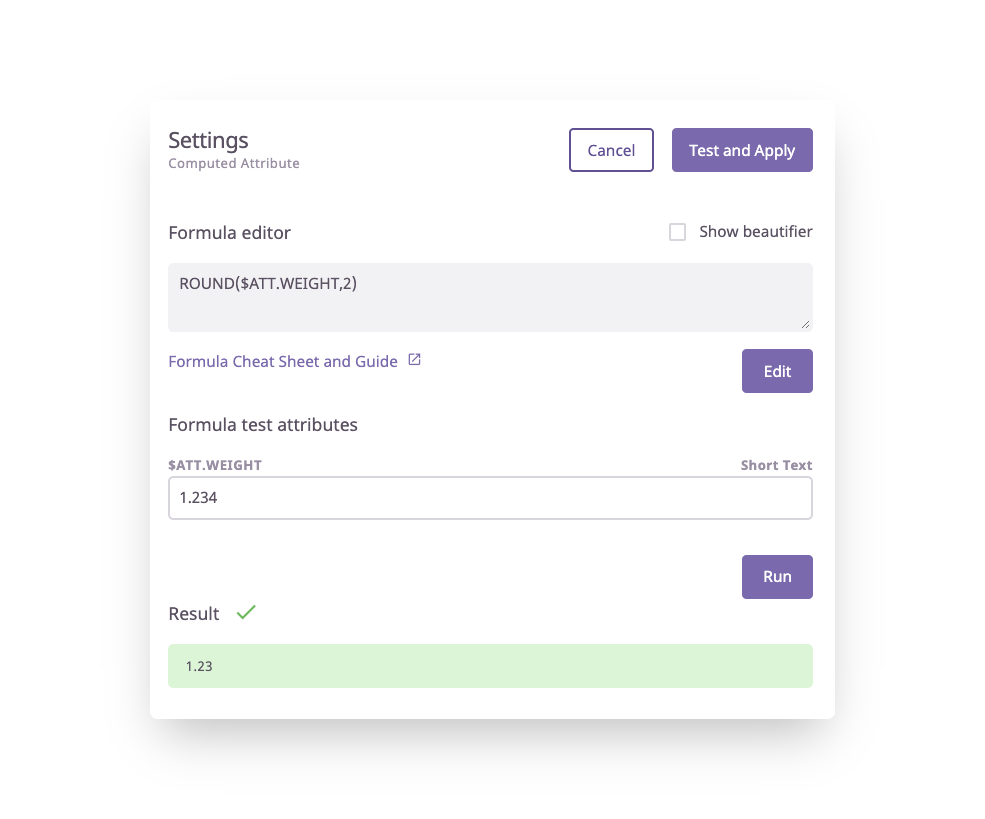# ROUND Operation

## How to use the ROUND operation for Computed Attributes

Definition

Example

Syntax Guide

Formula In Use

### Definition

The ROUND operation will round a number to a specified decimal place using normal rounding rules.

### Example

ROUND(15.9952, 2)

Result:
16.00

### Syntax Guide

ROUND(value, [places])

value - The value to round to places number of places

places - [ OPTIONAL - 0 by default ] - The number of decimal places to which to round

ℹ️ Places may be negative, in which case value is rounded at the specified number of digits to the left of the decimal point.

### Formula In Use

ROUND(\$ATT.WEIGHT,2)

This formula tells the system to round any values within the Weight attribute to two decimal places.### What's Next?

If you have any questions just click on the chat box in the bottom-right corner and we'll be happy to answer them...

and please let us know 👇